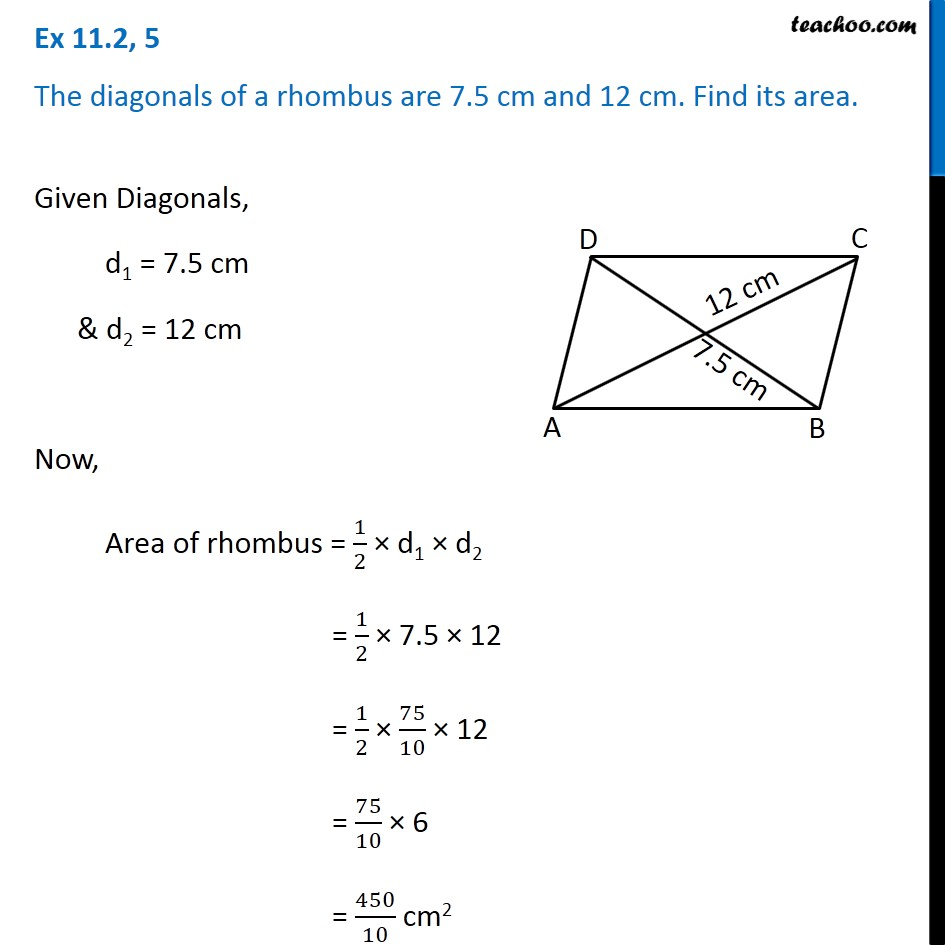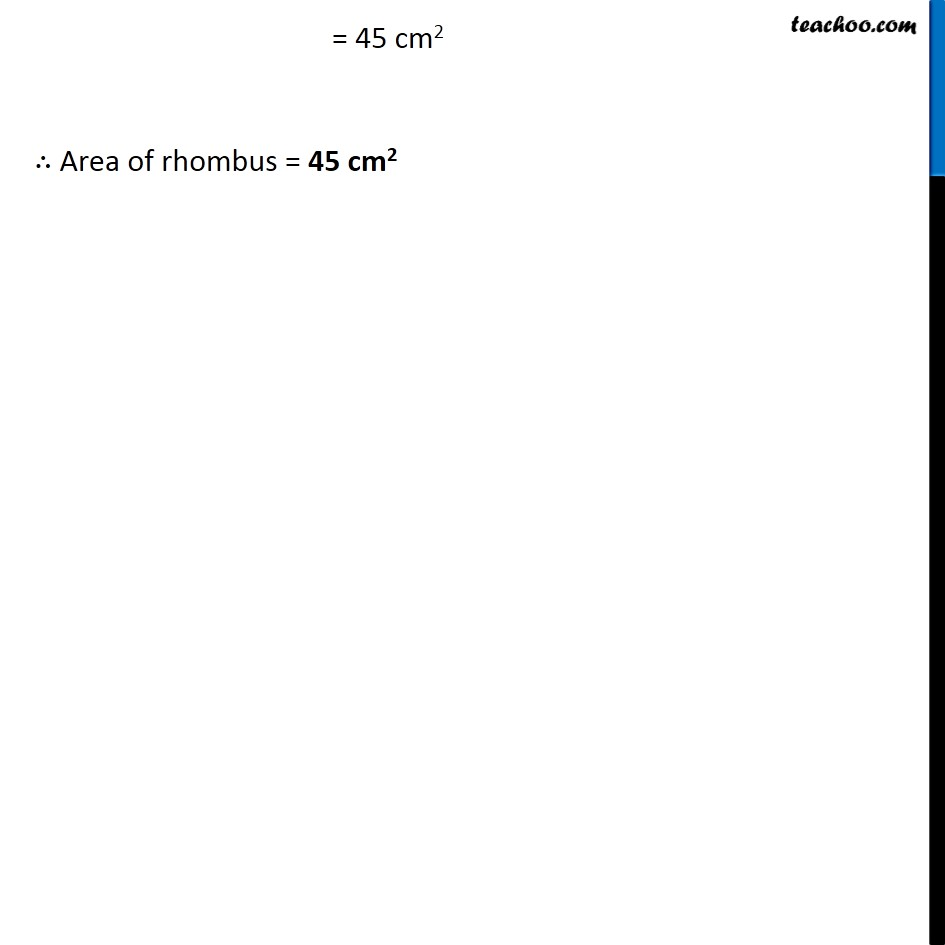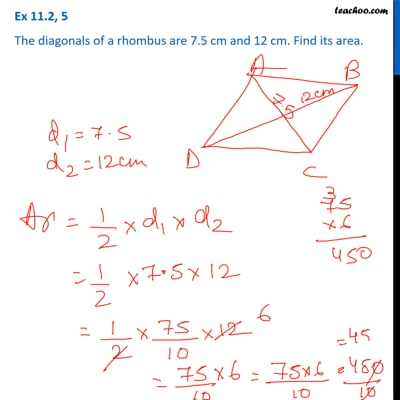Ex 11.2

Chapter 11 Class 8 Mensuration
Serial order wiseThis video is only available for Teachoo black users

Get live Maths 1-on-1 Classs - Class 6 to 12

### Transcript

Ex 11.2, 5 The diagonals of a rhombus are 7.5 cm and 12 cm. Find its area. Given Diagonals, d1 = 7.5 cm & d2 = 12 cm Now, Area of rhombus = 1/2 × d1 × d2 = 1/2 × 7.5 × 12 = 1/2 × 75/10 × 12 = 75/10 × 6 = 450/10 cm2 = 45 cm2 ∴ Area of rhombus = 45 cm2